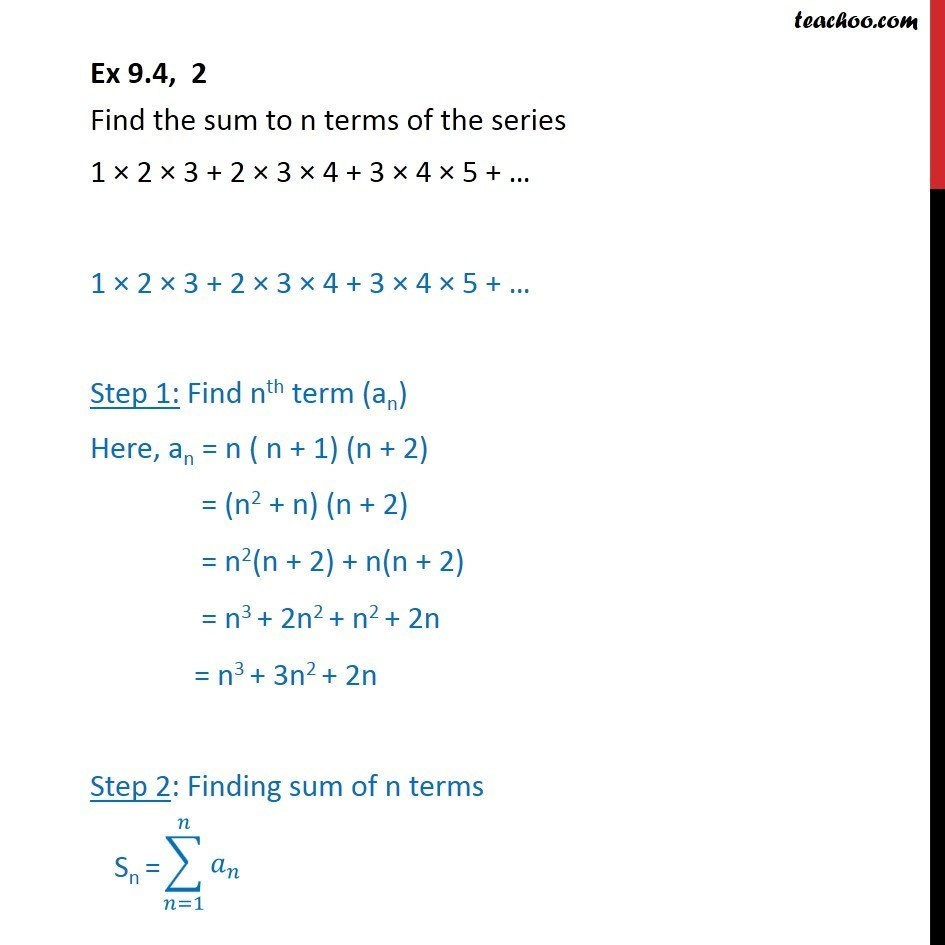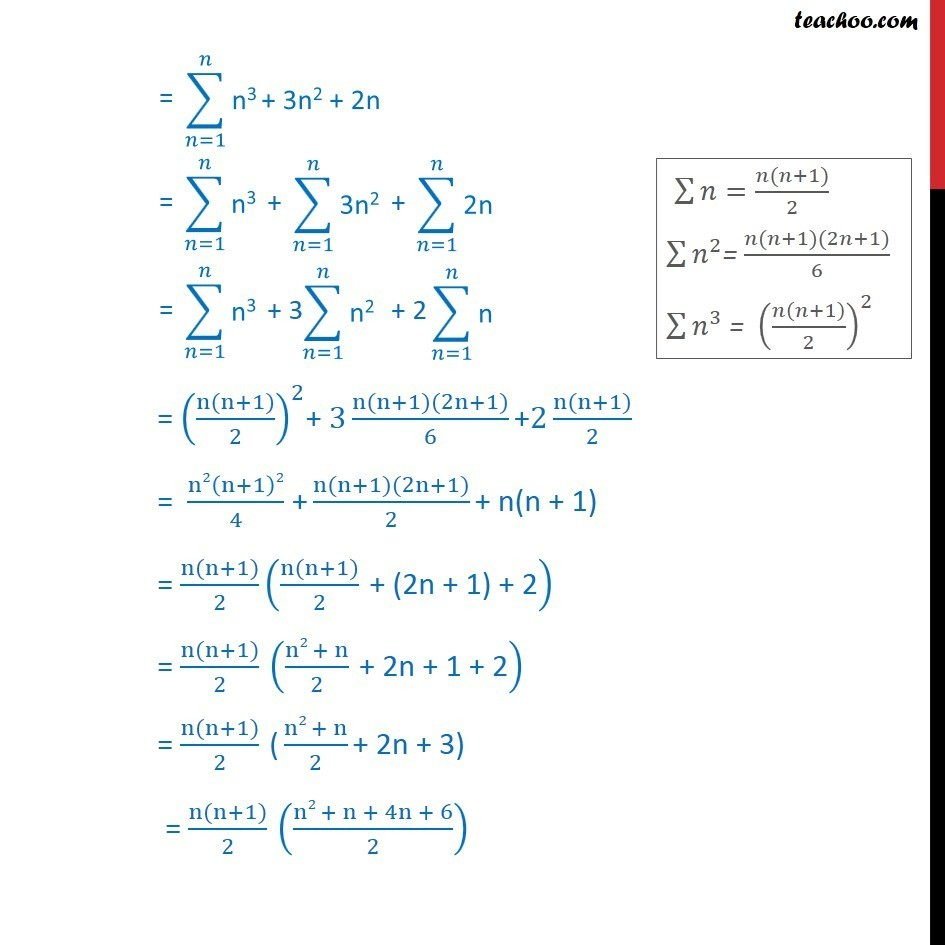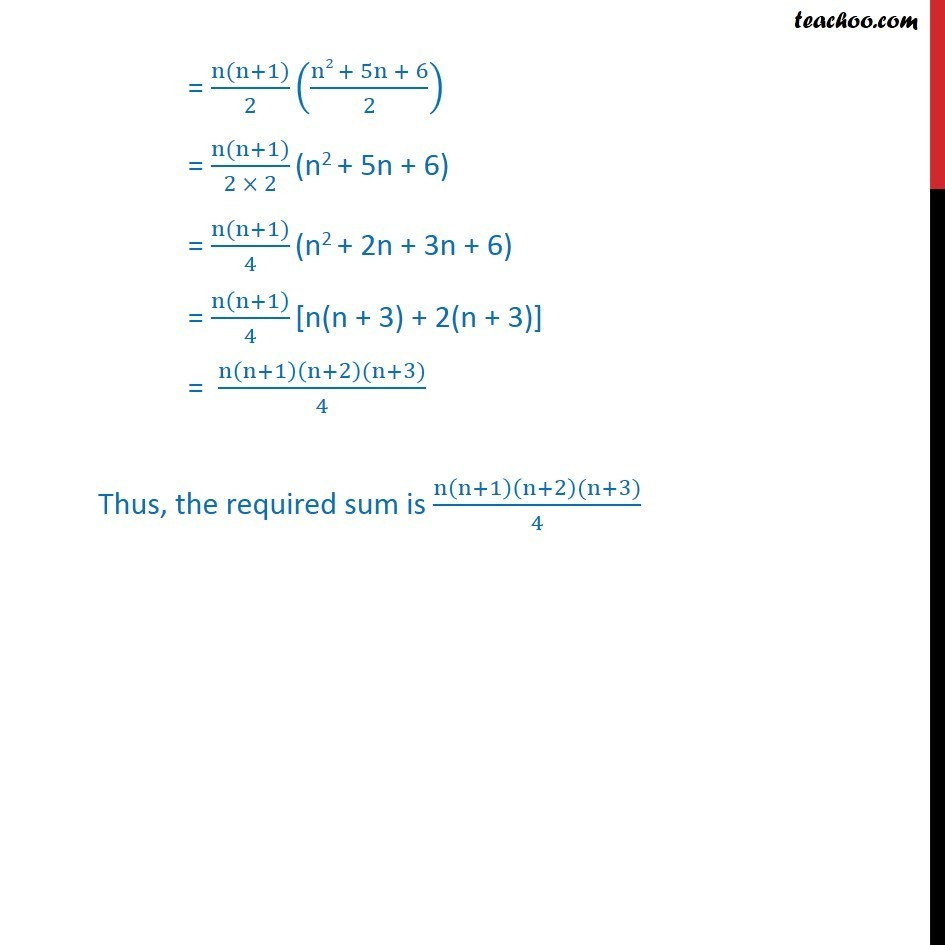1. Chapter 9 Class 11 Sequences and Series (Term 1)
2. Serial order wise
3. Ex 9.4

Transcript

Ex 9.4, 2 Find the sum to n terms of the series 1 × 2 × 3 + 2 × 3 × 4 + 3 × 4 × 5 + … 1 × 2 × 3 + 2 × 3 × 4 + 3 × 4 × 5 + … Step 1: Find nth term (an) Here, an = n ( n + 1) (n + 2) = (n2 + n) (n + 2) = n2(n + 2) + n(n + 2) = n3 + 2n2 + n2 + 2n = n3 + 3n2 + 2n Step 2: Finding sum of n terms = ((n(n+1))/2)^2+ 3 (n(n+1)(2n+1))/6 +2 (n(n+1))/2 = n2(n+1)2/4 + (n(n+1)(2n+1))/2 + n(n + 1) = (n(n+1))/2 ((n(n+1))/2 " + (2n + 1) + 2" ) = (n(n+1))/2 ((n2 + n)/2 " + 2n + 1 + 2" ) = (n(n+1))/2 ( (n2 + n)/2 + 2n + 3) = (n(n+1))/2 ((n2 + n + 4n + 6)/2) = (n(n+1))/2 ((n2 + 5n + 6)/2) = (n(n+1))/(2 × 2) (n2 + 5n + 6) = (n(n+1))/4 (n2 + 2n + 3n + 6) = n(n+1)/4 [n(n + 3) + 2(n + 3)] = (n(n+1)(n+2)(n+3))/4 Thus, the required sum is (n(n+1)(n+2)(n+3))/4

Ex 9.4

Chapter 9 Class 11 Sequences and Series (Term 1)
Serial order wise